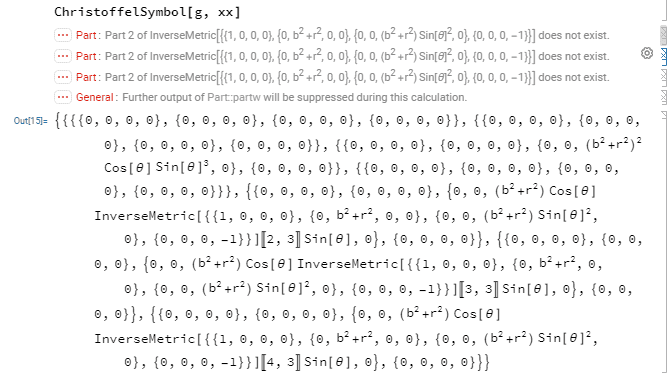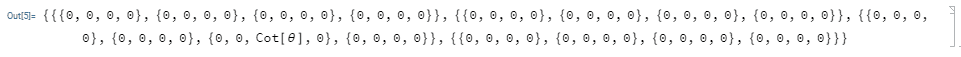# Learning how to compute Christoffel symbols using Mathematica

• Mathematica
• JD_PM

#### JD_PM

TL;DR Summary
I want to compute the Christoffel-symbol for a given metric.
I am using the code provided by Artes here, but I am missing something.

The Chrisfoffel-symbol formula is

$$\Gamma^{\mu}_{\phantom{\mu}\nu\sigma}=\frac{1}{2}g^{\mu\alpha}\left\{\frac{\partial g_{\alpha\nu}}{\partial x^{\sigma}}+\frac{\partial g_{\alpha\sigma}}{\partial x^{\nu}}-\frac{\partial g_{\nu\sigma}}{\partial x^{\alpha}}\right\}\quad$$

The metric is given to be

$$g_{\mu \nu} = \begin{pmatrix} 1 & 0 & 0 & 0 \\ 0 & r^2+b^2 & 0 & 0 \\ 0 & 0 & (r^2+b^2)\sin^2(\theta) & 0 \\ 0 & 0 & 0 & -1 \end{pmatrix}$$

The provided solution is:

$$\Gamma^{1}_{22}=-r$$

$$\Gamma^{1}_{33}=-r\sin^2(\theta)$$

$$\Gamma^{2}_{21}=\frac{r}{b^2+r^2}$$

$$\Gamma^{2}_{33}=-\cos(\theta)\sin(\theta)$$

$$\Gamma^{3}_{31}=\frac{r}{b^2+r^2}$$

$$\Gamma^{3}_{32}=\cot(\theta)$$

The code I'm using is

Code:
    xx = {t, x, \[Theta], \[Phi]};

g  = { {1,0,0,0},
{0,r^2+b^2,0,0},
{0,0,(r^2+b^2)Sin[\[Theta]]^2,0},
{0,0,0,-1}};

ChristoffelSymbol[g_, xx_] :=
Block[{n, ig, res},
n = 4; ig = InverseMetric[ g];
res = Table[(1/2)*Sum[ ig[[i,s]]*(-D[ g[[j,k]], xx[[s]]] +
D[ g[[j,s]], xx[[k]]]
+ D[ g[[s,k]], xx[[j]]]),
{s, 1, n}],
{i, 1, n}, {j, 1, n}, {k, 1, n}];
Simplify[ res]
]

But I getWhat am I missing? Besides, I'd like to learn how could I display the answer (once I know how to actually get it of course).

Any help is appreciated.

Thank you•etotheipi

What is InverseMetric?

•JD_PM
Oh I did not define the InverseMetric! Thanks for pointing it out.

I now have

Code:
xx = {t, x, \[Theta], \[Phi]};

g = { {1,0,0,0},
{0,r^2+b^2,0,0},
{0,0,(r^2+b^2)Sin[\[Theta]]^2,0},
{0,0,0,-1}};

InverseMetric=Simplify[Inverse[g]]

ChristoffelSymbol[g_, xx_] :=
Block[{n, ig, res},
n = 4; ig = InverseMetric;
res = Table[(1/2)*Sum[ ig[[i,s]]*(-D[ g[[j,k]], xx[[s]]] +
D[ g[[j,s]], xx[[k]]]
+ D[ g[[s,k]], xx[[j]]]),
{s, 1, n}], {i, 1, n}, {j, 1, n}, {k, 1, n}];
Simplify[ res]
]
ChristoffelSymbol[g, xx]

I now getSo apparently I only get ##\Gamma^3_{32}##

I found an alternative here

Code:
Clear [coord, metric, inversemetric, affine]

n = 4;
coord = {t, x, y,z};

metric = { {1,0,0,0}, {0,r^2+b^2,0,0}, {0,0,(r^2+b^2)Sin[\[Theta]]^2,0},
{0,0,0,-1}};

inversemetric = Simplify[Inverse[metric]];

affine :=
affine = Simplify[
Table[(1/2)*
Sum[inversemetric[[i,
s]]*(D[metric[[s, j]], coord[[k]]] +
D[metric[[s, k]], coord[[j]]] -
D[metric[[j, k]], coord[[s]]]), {s, 1, n}], {i, 1, n}, {j,
1, n}, {k, 1, n}]];

listaffine :=
Table[If[UnsameQ[affine[[i, j, k]],
0], {ToString[\[CapitalGamma][i - 1, j - 1, k - 1]],
affine[[i, j, k]]}], {i, 1, n}, {j, 1, n}, {k, 1, j}];
TableForm[Partition[DeleteCases[Flatten[listaffine], Null], 2],
TableSpacing -> {2, 2}]

I am struggling now in how to call out the specific Christoffel symbols correctly; I am trying

Code:
affine[[3,3,2]]

But I get zero instead of ##\cot(\theta)##; the same happens to me with other non-zero terms.

•Ishika_96_sparkles and JD_PM
OK I understand how to do it now!(more details)

My issue was in how to call out the function. Let's go step by step:

1) Define the Christoffel symbol function:

Code:
    ChristoffelSymbol[g_, xx_] :=
Block[{n, ig, res}, n = Length[xx]; ig = Inverse[g];
res = Table[(1/2)*
Sum[ig[[i, s]]*(-D[g[[j, k]], xx[[s]]] + D[g[[j, s]], xx[[k]]] +
D[g[[s, k]], xx[[j]]]), {s, 1, n}], {i, 1, n}, {j, 1, n}, {k,
1, n}];
Simplify[res]]

2) Define the coordinates and the components of the metric wrt the coordinate basis

Code:
    (* The coordinates *)
xx = {r, \[Theta], \[Phi], t};

(* The metric *)
g = {{1, 0, 0, 0}, {0, r^2 + b^2, 0, 0}, {0,
0, (r^2 + b^2) Sin[\[Theta]]^2, 0}, {0, 0, 0, -1}};

3) Relabel the Christoffel symbol function so that you can call out specific Christoffel symbols

Code:
    sol = ChristoffelSymbol[g, xx] (* This calls the function! *);

sol[[1, 2, 2]](*for instance*)
(* -r *)

Gen. Relativity...•